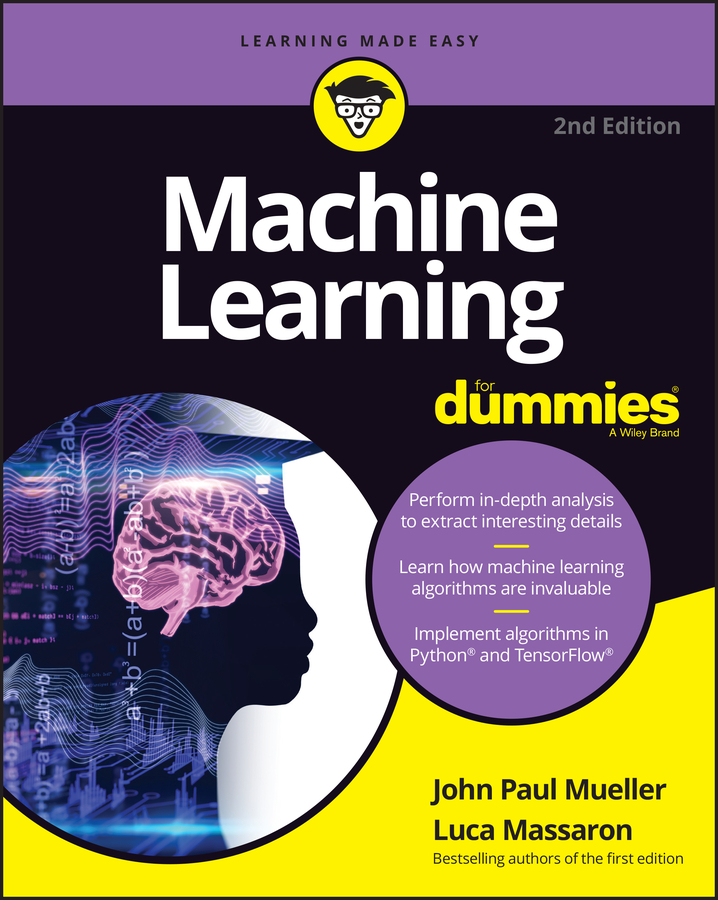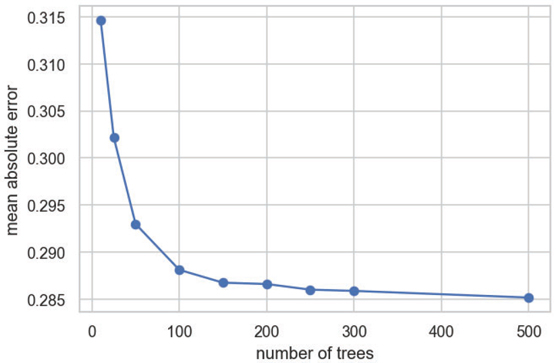##### Machine Learning For DummiesImproving a decision tree by replicating it many times and averaging results to get a more general solution sounded like such a good idea that it spread, and both academics and practitioners derived various solutions. When the problem is a regression, the technique averages results from the ensemble. However, when the trees deal with a classification task, the technique can use the ensemble as a voting system, choosing the most frequent response class as an output for all its replications. The following discussion is about how using trees for an ensemble creates a superior solution.

## Creating the Random Forests ensemble

When using an ensemble for regression, the standard deviation, calculated from all the ensemble’s estimates for an example, can provide you with an estimate of how confident you can be about the prediction. The standard deviation shows how good the mean of the estimates is. For classification problems, the percentage of trees predicting a certain class is indicative of the level of confidence in the prediction, but you can’t use it as a probability estimate because it’s the outcome of a voting system.

Deciding on how to compute the solution of an ensemble happened quickly; finding the best way to replicate the trees in an ensemble required more research and reflection. The first solution is pasting, that is, sampling a portion of your training set.

Initially proposed by Leo Breiman, pasting reduces the number of training examples, which can become a problem for learning from complex data because you get fewer examples to feed to the learning algorithm. It shows its usefulness by reducing the learning sample noise (sampling fewer examples reduces the number of outliers and anomalous cases).

After pasting, Professor Breiman also tested the effects of bootstrap sampling (sampling with replacement), which not only leaves out some noise (when you bootstrap, on average you leave out 37 percent of your initial example set) but also, thanks to sampling repetition, creates more variation in the ensembles, improving the results. This technique is called bagging (also known as bootstrap aggregation).

In bootstrapping, you sample the examples from a set to create a new set, allowing the code to sample the examples multiple times. Therefore, in a bootstrapped sample, you can find the same example repeated from one to many times.

Breiman noticed that results of an ensemble of trees improved when the trees differ significantly from each other (statistically, we say that they’re uncorrelated), which leads to the last technique of transformation—the creation of mostly uncorrelated ensembles of trees using different subsets of features.

The law of large numbers works because you make many independent trials of an event (for example, testing whether a coin is loaded on one side) and when you count the distribution of trials, you get the correct probability distribution of the event. Similarly, when you create a forest of decision trees, if they are independent from each other and therefore don’t make the same errors, you get estimates that, put together, are more correct. Breiman found out that decision trees become independent from each other if you randomize them by sampling both on training examples and on used features. This sampling approach performs predictions better than bagging. The transformation tweak samples both features and examples. Breiman, in collaboration with Adele Cutler, named the new ensemble Random Forests (RF).

Random Forests is a trademark of Leo Breiman and Adele Cutler. For this reason, open source implementations often have different names, such as `RandomForestClassifier` in Python’s Scikit-learn.

RF is a classification (naturally multiclass) and regression algorithm that uses a large number of decision tree models built on different sets of bootstrapped examples and subsampled features. Its creators strove to make the algorithm easy to use (little preprocessing and few hyper-parameters to try) and understandable (the decision tree basis) that can democratize the access of machine learning to nonexperts. In other words, because of its simplicity and immediate usage, RF can allow anyone to apply machine learning successfully.

The algorithm works through a few repeated steps:

1. Bootstrap the training set multiple times. The algorithm obtains a new set to use to build a single tree in the ensemble during each bootstrap.
2. Randomly pick a partial feature selection in the training set to use for finding the best split feature every time you split the sample in a tree.
3. Create a complete tree using the bootstrapped examples. Evaluate new subsampled features at each split. Don’t limit the full tree expansion to allow the algorithm to work better.
4. Compute the performance of each tree using examples you didn’t choose in the bootstrap phase (out-of-bag estimates, or OOB). OOB examples provide performance metrics without cross-validation or using a test set (equivalent to out-of-sample).
5. Produce feature importance statistics and compute how examples associate in the tree’s terminal nodes.
6. Compute an average or a vote on new examples when you complete all the trees in the ensemble. Declare for each of them the average estimate or the winning class as a prediction.
All these steps reduce the variance of the predictions at the expense of some increase of bias (because you use fewer features simultaneously). The solution builds each tree to its maximum possible extension, thus allowing a fine approximation of even complex target functions. Moreover, you increase the chance that each tree in the forest is different from the others because it’s built by fitting different samples. It’s not just a matter of building on different bootstrapped example sets: Each split taken by a tree is strongly randomized—the solution considers only a feature from a set defined by a random selection. Consequently, even if an important feature dominates the others in terms of predictive power, the times a tree doesn’t contain the selection allows the tree to find different ways of developing its branches and terminal leaves in an effective way.

The main difference with bagging is this opportunity to limit the number of features to consider when splitting the tree branches. If the number of selected features is small, the complete tree will differ from others, thus adding uncorrelated trees to the ensemble. On the other hand, if the selection is small, the bias increases because the fitting power of the tree is limited. As always, determining the right number of features to consider for splitting requires that you use cross-validation or OOB estimate results.

There is a variant of RF called Extremely Randomized Trees (ERT) that is even more randomized because it not only randomly picks the features for the splits but also randomly decides the splits. In this version, more variance is traded for more bias and, in some data problems, it may work better than RF. In addition, it is always faster because ERT requires fewer computations. No problem arises in growing a high number of trees in the ensemble. The more trees, the more precise and stable your estimates will become. You do need to consider the cost of the computational effort; completing a large ensemble takes a long time. RF is an algorithm that you can run in parallel on multiple CPU processors.

Each tree in the RF ensemble is independent from the others (after all, they should be uncorrelated), which means that you can build each tree in parallel to the others. Given that all modern computers have multiprocessor and multithread functionality, they can perform computations of many trees at the same time, which is a real advantage of RF over other machine learning algorithms.

## Demonstrating the RF algorithm

A simple demonstration conveys how an RF algorithm can solve a simple problem using a growing number of trees. This example uses the wine quality dataset, which models wine preferences by data mining physicochemical (physical and chemical) properties, combining both white and red wines. This dataset is described in “Modeling wine preferences by data mining from physicochemical properties,” by P. Cortez, A. Cerdeira, F. Almeida, T. Matos, and J. Reis, in Decision Support Systems (Elsevier, 47(4): 547-553, 2009). The data associates physicochemical tests (alcohol concentration, density, acidity and so on) on some Portuguese wines with the quality evaluation of experts. The dataset offers the opportunity to treat it both as a regression and a classification problem. This example works it out as a regression problem. It begins by loading the data and creating both train and test sets.
```import numpy as np
import pandas as pdtry:
import matplotlib.pyplot as plt
import seaborn as sns
sns.set(style='whitegrid', palette='deep',
font='sans-serif')
except:
import matplotlib.pyplot as pltfilename = 'https://github.com/lmassaron/datasets/'
train = (wine.groupby('quality')
.apply(lambda x: x.sample(frac=.7))
.reset_index(drop=True))
test = wine[~wine.index.isin(train.index)]X_train = train.iloc[:,1:]
y_train = train.iloc[:,0]
X_test = test.iloc[:,1:]
y_test = test.iloc[:,0]
```
After loading the dataset from the book’s Internet repository at GitHub (see the Introduction for details), the code uses pandas to apply stratified sampling (so that the response variable, `quality`, is distributed equally in the train and test data) in order to reserve 30 percent of observations as the test set.

In pandas, you can sample from a `DataFrame `using the `sample()` method, but that is just random sampling. If you need to stratify by a feature to assure that you sample that feature in the right proportions, you first group data based on that feature using the `groupby()` method and then you apply the sample method using the `apply()` method and a lambda function. For example, to sample 80 percent of df based on a feature: `df.groupby('feature').apply(lambda x: x.sample(0.8))`. The next step is to model the problem as shown here:

```from sklearn.metrics import mean_absolute_error
from sklearn.ensemble import RandomForestRegressorseries = [10, 25, 50, 100, 150, 200, 250, 300, 500]test_scores = list()
for param in series:
rf = RandomForestRegressor(n_estimators=param,
max_depth=14, random_state=42, jobs=-1)
rf.fit(X_train, y_train)
preds = rf.predict(X_test)
test_scores.append(mean_absolute_error(y_test, preds))
```

The example begins by importing functions and classes from Scikit-learn: `mean_absolute_error`, for measuring and `RandomForestRegressor` for modelling the problem. The last item is Scikit-learn’s implementation of Random Forests for regression problems. After defining some possible values for the `n_estimator` parameter, which specifies the number of decision trees in the RF, the code iterates the values and tests each one by first training the regressor and then evaluating the result on the test set.

To make the example run faster, the code sets the `n_jobs` parameter to `–1`, allowing the algorithm to use all available CPU resources. This setting may not work on some computer configurations, because the computer now uses all resources to train the model. As an alternative, you can set the parameter to `-2`, thus leaving one processor free for other tasks.

After completing the computations, the code outputs a plot that reveals how the Random Forests algorithm converges to a good accuracy after building a few trees, as shown. It also shows that adding more trees isn’t detrimental to the results because the error tends to stabilize and even improve a little after reaching a certain number of trees.
```import matplotlib.pyplot as pltfig, ax = plt.subplots(dpi=120)
plt.plot(series, test_scores, '-o')
plt.xlabel('number of trees')
plt.ylabel('mean absolute error')
plt.show()
```Seeing the accuracy of ensembles of different sizes.